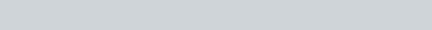# Pump efficiency

How efficient is your pump? 7-2

A few years ago, efficiency became “the name of the game”. Automotive companies advertised “miles per gallon (liters per 100 kilometers) information in their advertisements and appliance manufacturers published kilowatt consumption numbers along with their pricing information.

Unfortunately high efficiency also means higher maintenance costs because you are required to maintain tighter tolerances and keep the flow passages smooth and free from obstructions. The demise of the double volute pump design in smaller size pumps, is a perfect example of the increase in mechanical seal problems as the efficiency of the volute pump was increased to satisfy consumer demand.

Maybe the “trade off” is acceptable as long as you are dealing with accurate numbers, but are you really doing that? Is the efficiency shown on the pump curve accurate? How was the data taken? What was included in the data, and more important, what was left out? As an example:

• Was the data generated on a dynamometer with a constant speed motor?
• Are you going to run at the same speed as shown on the performance curve, or are you running with an induction motor that slips 2% to 5% and you are not sure of the actual speed? Horse power (K.W.) varies as the cube of the change in speed at the best efficiency point, so a small variation in speed can make a big difference in efficiency.
• Was the published efficiency data generated with a seal or packing in the stuffing box? The type of packing or seal used can alter the load they consume.
• Was there an elbow at the suction of the pump?
• Was the inside of the volute polished or coated with a low friction material when the test was made?
• How were the bearings lubricated, and were all of the losses considered in the published numbers?
• The final numbers will vary with the motor efficiency, and that will vary with the load on the motor.

If you would like to keep the pump salesman honest, take the data from his pump curve and then make the following calculation:

In inch sizes : GPM x TDH / 3960 = WHP

• GPM = Gallon per minute at the best efficiency point
• TDH = Total discharge head (measured in feet), as shown on the pump curve&emdash; at the best efficiency point)
• WHP = Water horse power, or the amount of horse power the pump is generating.If we refer to the above pump curve, and insert the numbers into our formula, we would get:in inch size: GPM x TDH / 3960 = 250 X 300 / 3960 = 18.9

You then divide this number by the efficiency shown on the pump curve:

18.9 / .60 = 31.5 horsepower required to generate the WHP. If this number is lower than the horsepower shown on the performance curves, the efficiency date is questionable. As an example:

If the performance curve showed a requirement for 40 Horse power, the actual efficiency would be 18.9 water horse power40 pump horsepower = .47 or 47% actual efficiency.

Doing the same thing in the metric system we would get:

• M3/ HR = Cubic meters per hour of capacity as measured at the best efficiency point on the pump curve.
• TDH = Total discharge head, in meters, at the best efficiency point.
• WKW = Water kilowatts of power being generated by the pump.

Referring to the above diagram, and putting in the numbers :

M3 / HR X TDH / 360 = 68 x 76 / 360 = 14.36 WKW. The curve shows a 60% efficiency so:

14.36 water kilowatts / 0.60 efficiency = 23.93 Kilowatts required. If this number is lower than shown on the pump performance curve, the efficiency of the plump is questionable. As an example:

If the pump performance curve showed a requirement for a 30 Kilowatt input, the actual efficiency would be:

14.36 water horse power / 30 Kilowatts required = 48 % actual efficiency.

The fact of the matter is that you seldom operate at the best efficiency point so the numbers become even more depressing. The point is that efficiency should only be one of the points taken into consideration when you purchase a centrifugal pump of a given head, material and capacity. Equally if not more important should be:

• The L3/D4 number of the shaft. Is the number below 60 in inch sizes or 2 in metric?
• What kind of mechanical seal is installed? Will it seal fugitive emissions?
• How are the bearings being lubricated?
• How are the bearings sealed? Will the seal damage the expensive shaft?
• How is the thrust bearing being retained? In operation the impeller thrusts towards the volute. Are you relying upon a simple snap ring?
• Is the pump a centerline design? It should be if the product you are pumping is greater than 200°F (100 C.)
• Is the bearing case vented to atmosphere? If it is, it will allow moisture to penetrate when the pump stops.
• Has a “C” or “D” frame adapter been installed to reduce alignment time?
• Can the wear rings or open impeller be easily adjusted to compensate for normal wear so that you can keep the efficiency you paid for?
• Can the seal compensate for thermal growth, or impeller adjustment?

You can save money by lowering operating costs (efficiency) or increasing the time between repairs (design). Be sure you consider both when you make your pump buying decision.

### Posted

• On February 17, 2018